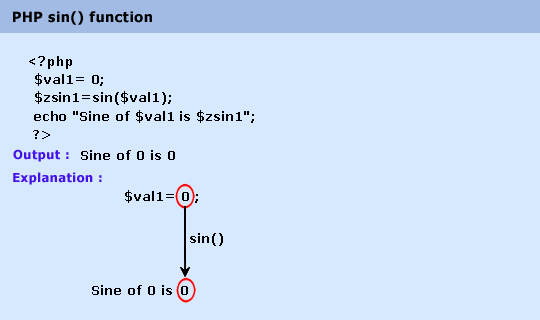# Education is not limited to just classrooms. It can be gained anytime, anywhere... - Ravi Ranjan (M.Tech-NIT)

## PHP : sin() function

Description

The sin() function is used to get the sine of a number.

Version

(PHP 4 and above)

Syntax

sin(num)

Parameter

Name

Description

Required /
Optional

Type

num

A value in radian.

Required

Foat.

Return value

The sine of num.

Value Type : Float.

Pictorial PresentationExample :

1. \$val1= 0;

2. \$val2=4;

3. \$val3=-4;

4. \$zsin1=sin(\$val1);

5. \$zsin2=sin(\$val2);

6. \$zsin3=sin(\$val3);

7. echo "Sine of \$val1 is \$zsin1
";

8. echo "Sine of \$val2 is \$zsin2
";

9. echo "Sine of \$val3 is \$zsin3
";

10. ?>

Output :

Sine of 0 is 0
Sine of 4 is -0.756802495308
Sine of -4 is 0.756802495308